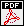# Theorems of Existence and Uniqueness of Generalized Solutions for One Class of Nonstationary Problems of Coupled Electroelasticity

Melnik (R.)V.N.

Soviet Mathematics, 35(4), 23-30, 1991 [Referenced also as: Izvestiya Vysshikh Uchebnykh Zavedenii (VUZ): Matematika, 35(4), 24-32 1991]

### Abstract:

The focus of this paper is the mathematical analysis of a general class of fully coupled models of dynamic electroelasticity. Such models have extensive applications and have been used by scientists and engineers as a key mathematical description for the ubiquitous phenomenon of piezoelectricity. Although models of this type have been known since Woldemar Voigt's works on piezoelectricity, dating back at least to 1910 when "Lerbuch der Kristallphysik" appeared, the mathematically rigorous proof of existence and uniqueness of solutions to such models remained absent in such a general non-stationary setting. In this paper, this missing link has been filled. With the use of the Faedo-Galerkin method, the existence, uniqueness and regularity of generalized solutions to the corresponding initial-boundary value problem have all been proved. The results have been demonstrated on a typical example based on the equation of motion of infinite, radially polarized hollow piezoelectric cylinders, coupled with the equation of electrostatics for dielectrics.